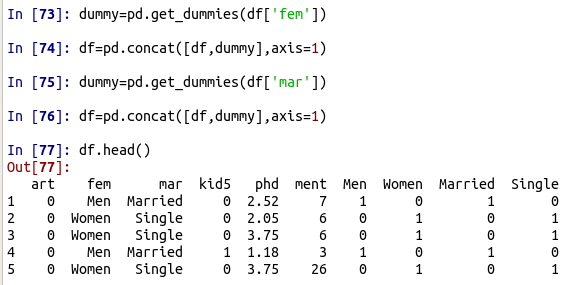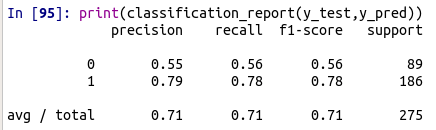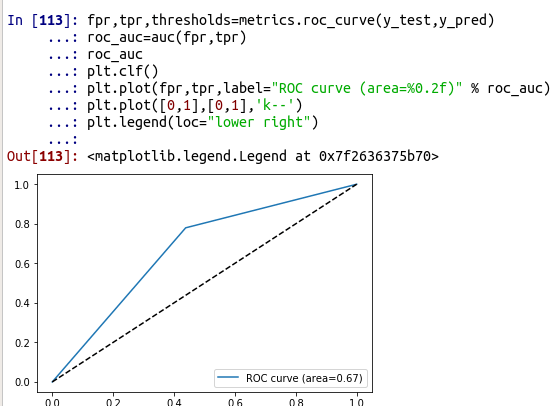# Linear Discriminant Analysis in Python

Linear discriminant analysis is a classification algorithm commonly used in data science. In this post, we will learn how to use LDA with Python. The steps we will for this are as follows.

1. Data preparation
2. Model training and evaluation

Data PreparationWe will be using the bioChemists dataset which comes from the pydataset module. We want to predict whether someone is married or single based on academic output and prestige. Below is some initial code.

```import pandas as pd
from pydataset import data
import matplotlib.pyplot as plt
from sklearn.discriminant_analysis import LinearDiscriminantAnalysis as LDA
from sklearn.model_selection import train_test_split
from sklearn.metrics import classification_report```

Now we will load our data and take a quick look at it using the .head() function.There are two variables that contain text so we need to convert these two dummy variables for our analysis the code is below with the output.Here is what we did.

1. We created the dummy variable by using the .get_dummies() function.
2. We saved the output in an object called dummy
3. We then combine the dummy and df dataset with the .concat() function
4. We repeat this process for the second variable

The output shows that we have our original variables and the dummy variables. However, we do not need all of this information. Therefore, we will create a dataset that has the X variables we will use and a separate dataset that will have our y values. Below is the code.

```X=df[['Men','kid5','phd','ment','art']]
y=df['Married']```

The X dataset has our five independent variables and the y dataset has our dependent variable which is married or not. We can not split our data into a train and test set.  The code is below.

`X_train,X_test,y_train,y_test=train_test_split(X,y,test_size=.3,random_state=0)`

The data was split 70% for training and 30% for testing. We made a train and test set for the independent and dependent variables which meant we made 4 sets altogether. We can now proceed to model development and testing

Model Training and Testing

Below is the code to run our LDA model. We will use the .fit() function for this.

```clf=LDA()
clf.fit(X_train,y_train)```

We will now use this model to predict using the .predict function

`y_pred=clf.predict(X_test)`

Now for the results, we will use the classification_report function to get all of the metrics associated with a confusion matrix.The interpretation of this information is described in another place. For our purposes, we have an accuracy of 71% for our prediction.  Below is a visual of our model using the ROC curve.Here is what we did

1. We had to calculate the roc_curve for the model this is explained in detail here
2. Next, we plotted our own curve and compared to a baseline curve which is the dotted lines.

A ROC curve of 0.67 is considered fair by many. Our classification model is not that great but there are worst models out there.

Conclusion

This post went through an example of developing and evaluating a linear discriminant model. To do this you need to prepare the data, train the model, and evaluate.